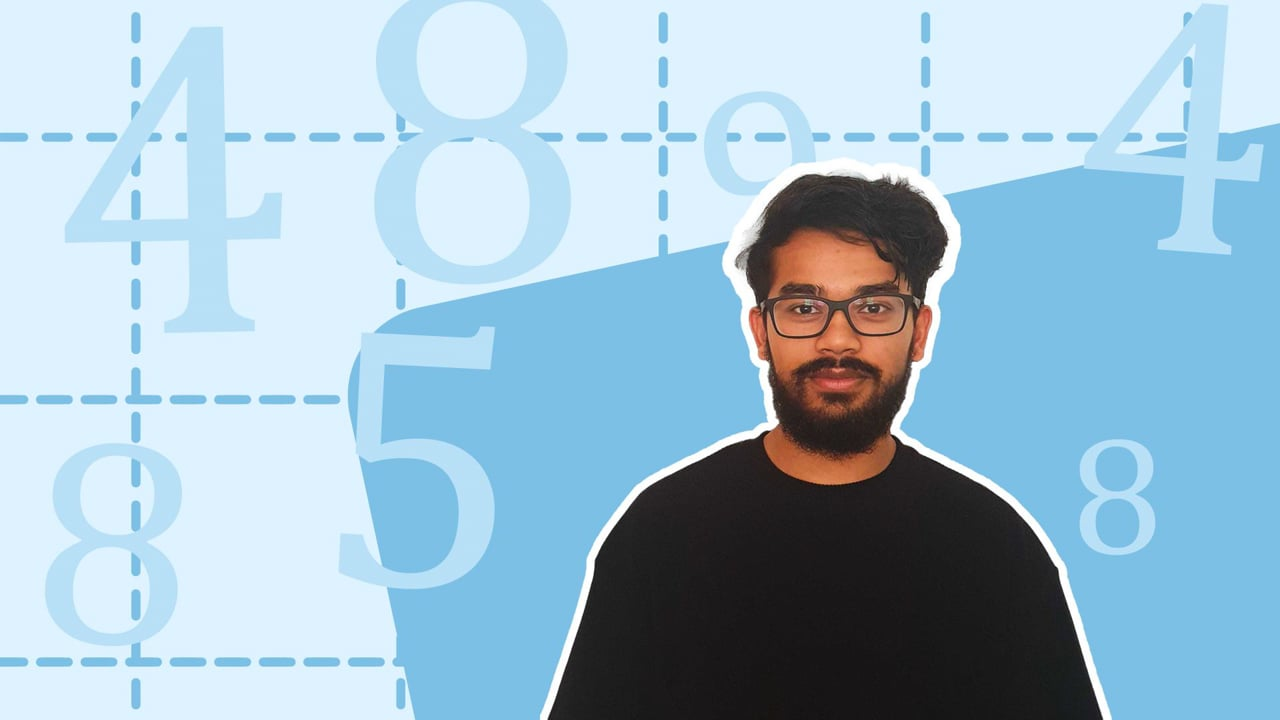Chapter OverviewMaths

Number and place value

Multiplication and division

Fractions

Measurement

Geometry - properties of shapes

Geometry - position and direction

Statistics

Maths

# Multiples and factors0%

Summary

# ​​Multiples and factors

## In a nutshell

A multiple is a number you get when you multiply a certain number by a whole number. A factor is a number that divides into a certain number exactly with no remainder.

## Multiples

Multiples are the numbers you get by multiplying a certain number by a whole number.  The times table displays the multiples of different numbers.

##### Example 1

What are the first $10$ multiples of $5$?

\begin{aligned} 5 \times 1 &=5 \\ 5 \times 2 &= 10 \\ 5 \times 3 &= 15 \\5 \times 4 &= 20 \\5 \times 5 &= 25 \\5 \times 6 &= 30 \\5 \times 7 &= 35 \\5 \times 8 &= 40 \\5 \times 9 &= 45 \\ 5 \times 10 &= 5 0\\\end{aligned}

$\underline{Multiples\space of\space 5 = 5,10,15,20,25,30,35,40,45,50.}$

​​

## Factors

Factors are numbers that divide into a certain number exactly with no remainder. Factors always come in pairs.

##### Example 2

What are the factors of $30$​?

\begin{aligned} &1 \times 30 &= 30 \\ &2 \times 15 &= 30 \\ &3 \times 10 &= 30 \\ &5 \times 6 &= 30 \end{aligned}

$\underline{Factors\space of\space30=1,2,3,5,6,10,15,30.}$​​

Note: All numbers are a factor of themselves and $1$

## Common factors

A common factor is a factor of two or more numbers which is the same.

##### Example 3

What are the common factors of $20$ and $8$?

Identify the factors of  $20$.

${Factors\space of\space20=1,2,4,5,10,20}$​​

Identify the factors of  $8$.

${Factors\space of\space8=1,2,4,8}$​​

Identify which factors are the same for $8$​ and $20$

$\underline{Factors\space of\space8 \space and\space 20 =1,2,4.}$​​

Note: All even numbers have a common factor of $2$.

FAQs

• Question: What do the time tables show?

Answer: A times table displays the multiples of a given number.

• Question: What are factors?

Answer: A factor is a number that divides into a certain number exactly with no remainder.

• Question: What are multiples?

Answer: A multiple is a number you get when you multiply a certain number by a whole number.

Theory

Exercises